## Colored Rhombic Octiamonds

If we color the eight unit edges of a rhombic octiamond with n colors, we get as many different pieces as if we color the four unit edges of a diamond with n^2 colors, because we can replace each combination of two colors out of n colors by a single color out of n^2 colors.But there are differences. If we clockwise pass the sides of the rhombic octiamond the color combinations of each side can be denoted by a-b. To get a match in a construction neighboring sides must be a-b and b-a, whereas the single colors at the sides of the diamonds must be equal. Furthermore there are octiamond pieces which are equivalent under reflection but the corresponding diamond pieces are not. This is also true vice versa. Click the numbers of pieces to get some constructions.

Colors Flip Type Number of Pieces Total Number
of Triangles
Constructions
n one-sided (n^8+n^4)/2
two-sided (n^8+3n^4)/4
2 one-sided 136 1088 34x16 parallelogram
two-sided 76 608 38x8 parallelogram
3 two-sided 1701 13608 3-fold parallelograms, hexagonal ring,
parallelograms, rhomb with similar hole

### Two Colors, One-sided Rhombic Octiamonds

Here is the parallelogram covered by the 136 pieces.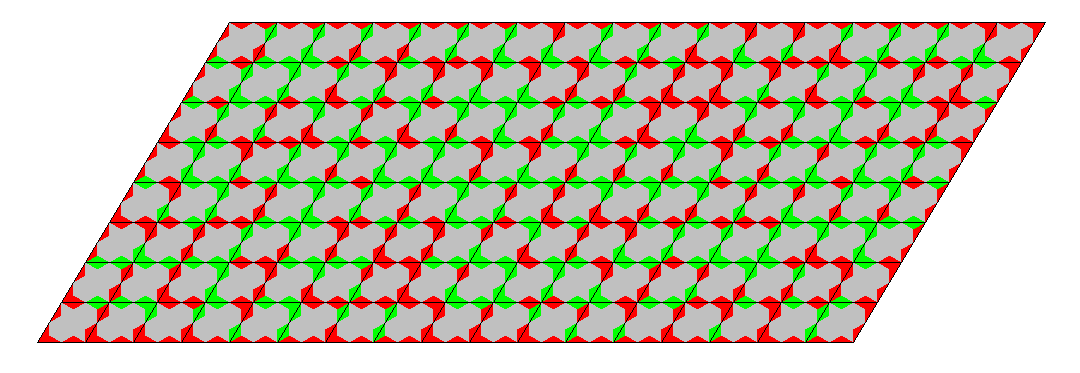The 68x8 parallelogram cannot be constructed. Rotate the pieces so that two sides are horizontal and the other ones have a positive slope. Only the horizontal sides can be used for the long sides of the parallelogram but the number of suitable pieces is too small.

### Two Colors, Two-sided Rhombic Octiamonds

Here is the 38x8 parallelogram. It isn't possible to derive the construction from a parallelogram made with four-colored diamonds because matching and flipping are different. Contrary to the one-sided case all sides of the pieces can contribute to the long borders and therefore we get enough same colored red edges.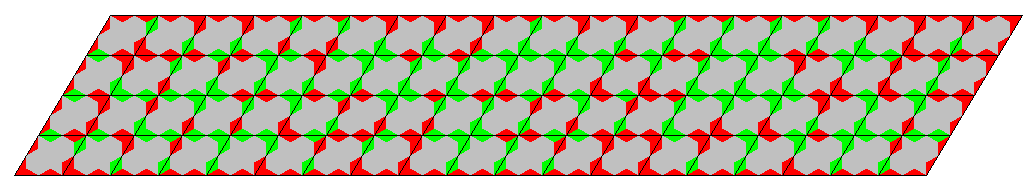### Three Colors, Two-sided Rhombic Octiamonds

Take a piece and shift colors from 1,2,3 to 2,3,1 or 3,2,1. Then we have 567 triples of pieces and we can make constructions using only one piece out of each triple. This way we get three congruent constructions each with a different border and all pieces taken. Possible parallelograms are 27x21, 63x9 and 81x7.

The 54x42 parallelogram. All three pictures are here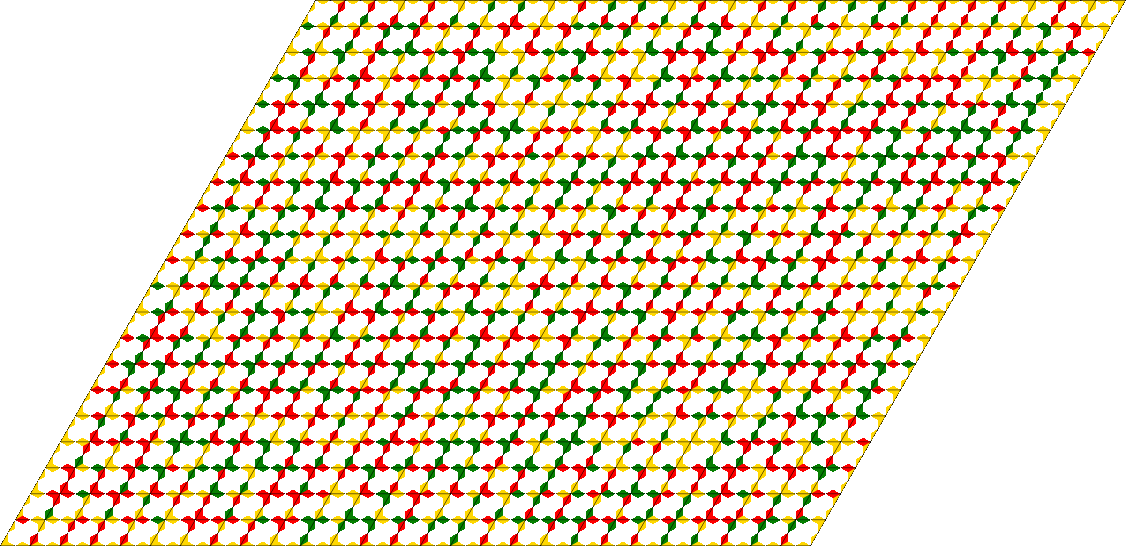The 126x18 parallelogram. All three pictures are here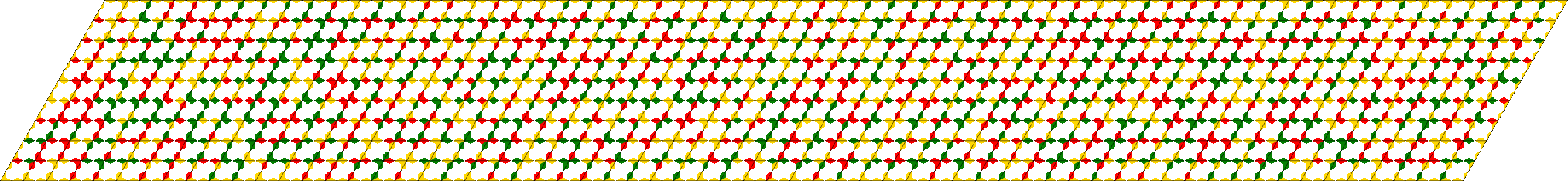The 162x14 parallelogram. All three pictures are hereAt last I tried to construct a hexagonal ring of size 48 with a hexagonal hole of size 6. Since the above method can't be applied the solution is much more difficult to get.A SVG-file for the construction is here

Taking diamonds and 9 single colors the rendering of the construction looks more colorful. You can see that matching colors are red-red, green-green, violet-violet, lime-teal, yellow-blue, aqua-fuchsia.A SVG-file for this picture is here

To show parallelograms of size 126x54 and 162x42 I used a different rendering.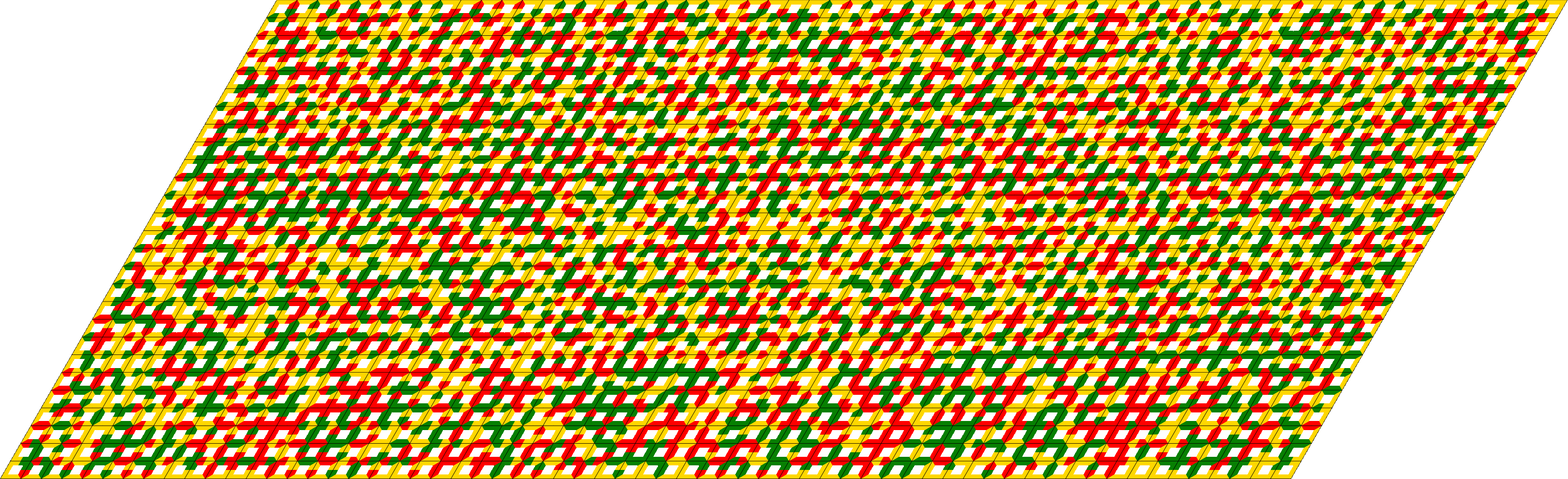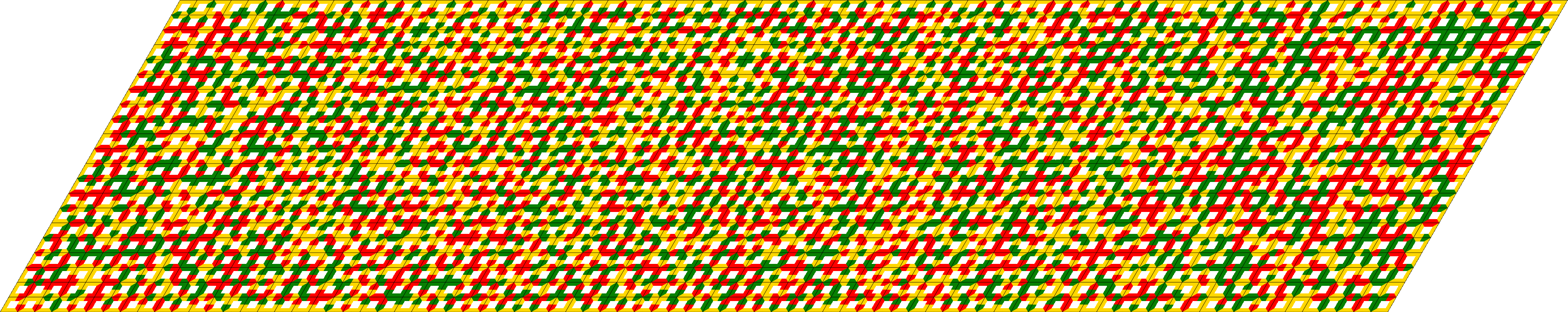If we omit the piece with all eight edge units colored yellow we can get a rhomb with a rhombic hole. The omitted piece may be placed in the center of the hole.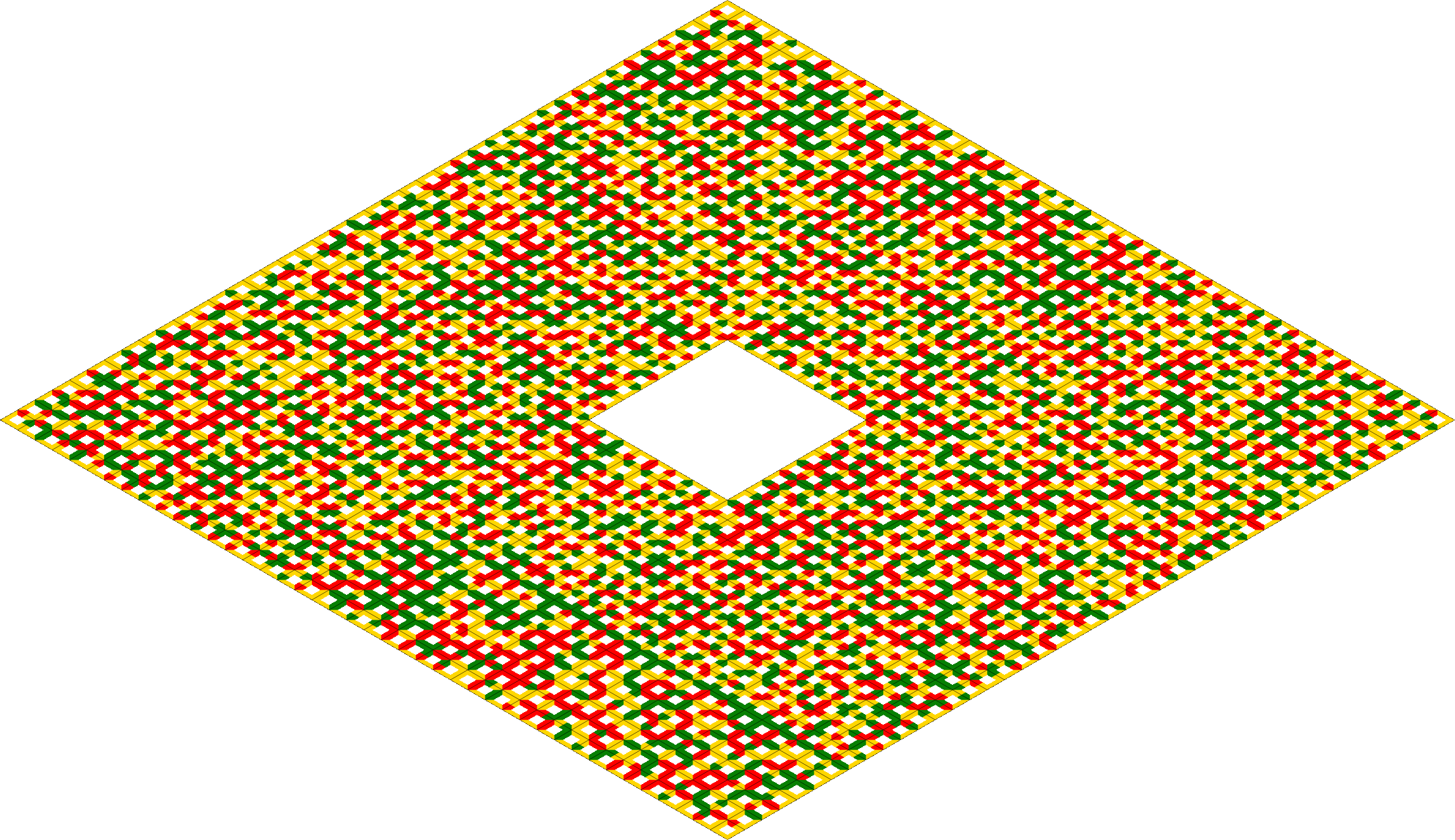Back
Home